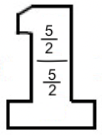### Home > MC1 > Chapter 9 > Lesson 9.1.2 > Problem9-17

9-17.

Calculate each of the following quotients.

1. $\frac { 2 } { 3 } \div \frac { 2 } { 5 }$

$\frac{\frac{2}{3}}{\frac{2}{5}} \cdot$$=$

1. $\frac { 5 } { 6 } \div \frac { 1 } { 12 }$

$10$
1. $3 \frac { 1 } { 8 } \div 2 \frac { 1 } { 2 }$
$\frac{\frac{25}{8}}{\frac{5}{2}}$